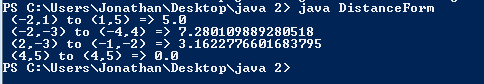# Assignment #93

## Code

```/// Name: Jonathan Stine
/// Period: 5
/// Program Name: Distance Formula
/// File Name: DistanceForm.java
/// Date Finished: 12/17/2016 (IN THE FUTURE MAN!!!)

public class DistanceForm
{
public static void main( String[] args )
{
// test the formula a bit
double d1 = distance(-2,1 , 1,5);
System.out.println(" (-2,1) to (1,5) => " + d1 );

double d2 = distance(-2,-3 , -4,4);
System.out.println(" (-2,-3) to (-4,4) => " + d2 );

System.out.println(" (2,-3) to (-1,-2) => " + distance(2,-3,-1,-2) );

System.out.println(" (4,5) to (4,5) => " + distance(4,5,4,5) );
}

public static double distance( int x1, int y1, int x2, int y2 )
{
double distance = Math.sqrt( (x2 - x1) * (x2 - x1) + (y2 - y1) * (y2 - y1) );
return distance;
}
}

```

### Code Output